Adding decimal numbers Worksheet AnswersCounting up to solve money word problems Worksheet Answers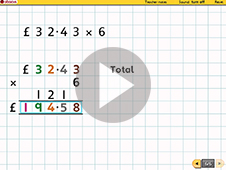Using short multiplication Worksheet Answers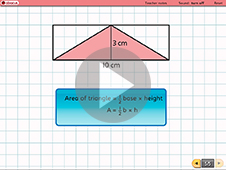Calculating the area of a triangle Worksheet Answers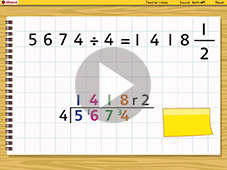Using short division Worksheet Answers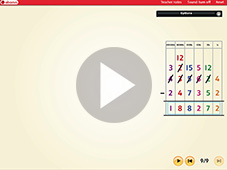Subtracting 6 and 7 digit numbers Worksheet Answers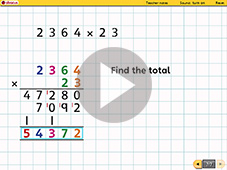Using long multiplication Worksheet Answers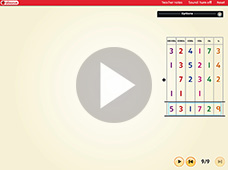Adding 5 and 6 digit numbers Worksheet Answers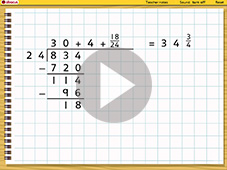Using long division Worksheet Answers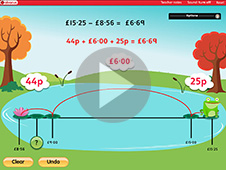Choosing an appropriate method to solve decimal subtractions Worksheet Answers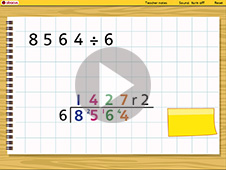Solve word problems using short division Worksheet Answers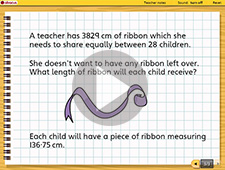Solve word problems using short division Worksheet Answers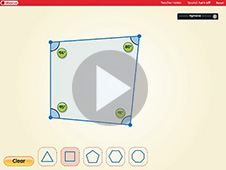Using a protractor and angle sums Worksheet Answers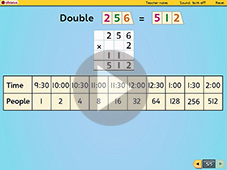Exploring a sequence Worksheet Answers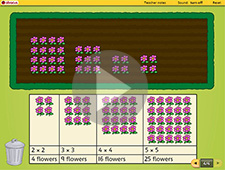Investigation : Square numbers Worksheet Answers